# Statistical distributions#

Plots of the distribution of at least one variable in a dataset. Some of these methods also compute the distributions.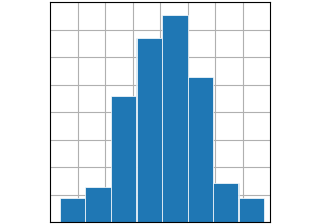hist(x)

hist(x)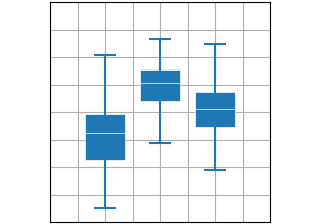boxplot(X)

boxplot(X)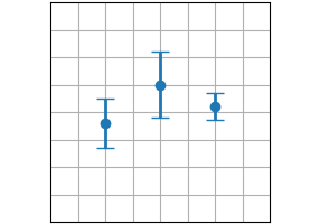errorbar(x, y, yerr, xerr)

errorbar(x, y, yerr, xerr)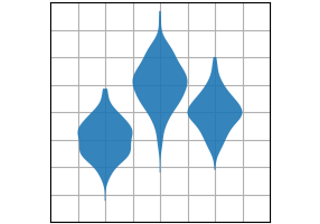violinplot(D)

violinplot(D)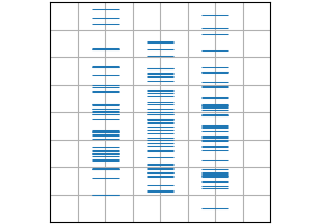eventplot(D)

eventplot(D)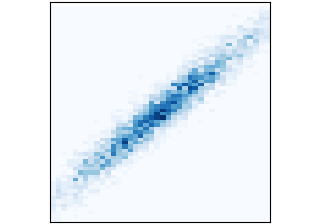hist2d(x, y)

hist2d(x, y)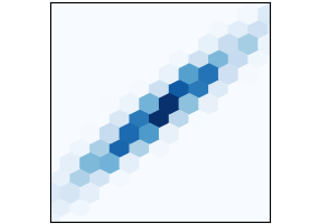hexbin(x, y, C)

hexbin(x, y, C)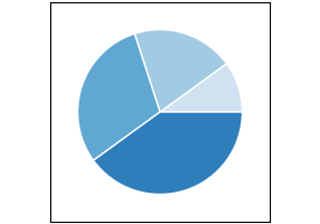pie(x)

pie(x)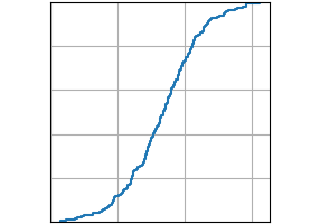ecdf(x)

ecdf(x)